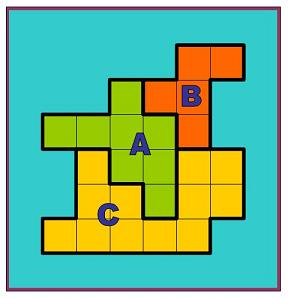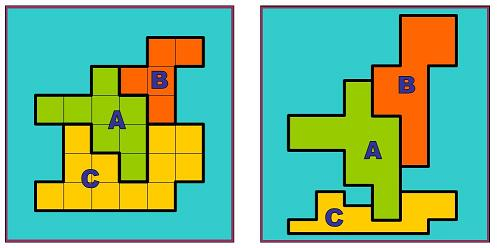#### You may also like### Golden Thoughts

Rectangle PQRS has X and Y on the edges. Triangles PQY, YRX and XSP have equal areas. Prove X and Y divide the sides of PQRS in the golden ratio.### At a Glance

The area of a regular pentagon looks about twice as a big as the pentangle star drawn within it. Is it?### Contact

A circular plate rolls in contact with the sides of a rectangular tray. How much of its circumference comes into contact with the sides of the tray when it rolls around one circuit?

# The Rescaled Map

#### Getting an Informed View

We use statistics to give ourselves an informed view on a subject of interest. This problem explores how to scale countries on a map to represent characteristics other than land area.

To see why this might be a valuable thing to do take a look at this site : https://worldmapper.org/

Let's start with a simple example of a three-country continent. The countries all share a border with each other and together are surrounded by sea.In this first view the areas of countries A, B and C are 8, 5, and 12 million sq.km

Your challenge is to complete a new view that expresses each country's population of 40, 36, and 24 million people respectively. You must retain enough of the shape and relative position for each country to be recognisable.

One way you might do this is given below - can you improve on it?

Let the ocean keep its proportion of the map.
39 squares were ocean in the first view, and the same area of the map must be ocean in the new view.

Country A has a land area of 8 million sq.km out of a total land area of 25 million sq.km, that's 32% of the land. Country A also has 40 million people from a total of 100 million, which is 40% of the total population.

That means that A needs to cover a larger area of the new map (1.25 times more to scale up from 32 to 40)

Similar calculations mean that the area of country B must scale up by 1.8 and the area of country C should be multiplied by 0.5 (half as much area)

The question now is how to apply these multipliers - here is the result of a stretching each country by its scale factor in the vertical direction (scaled version on the right).• Can you see how this method works?